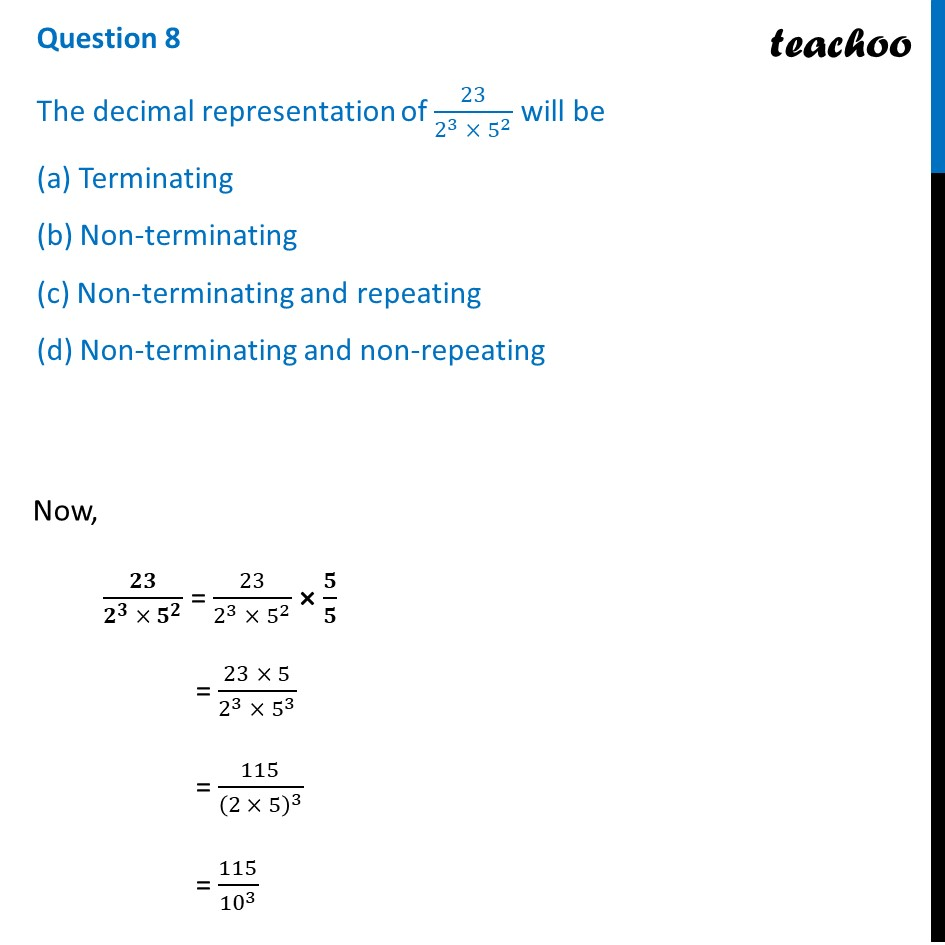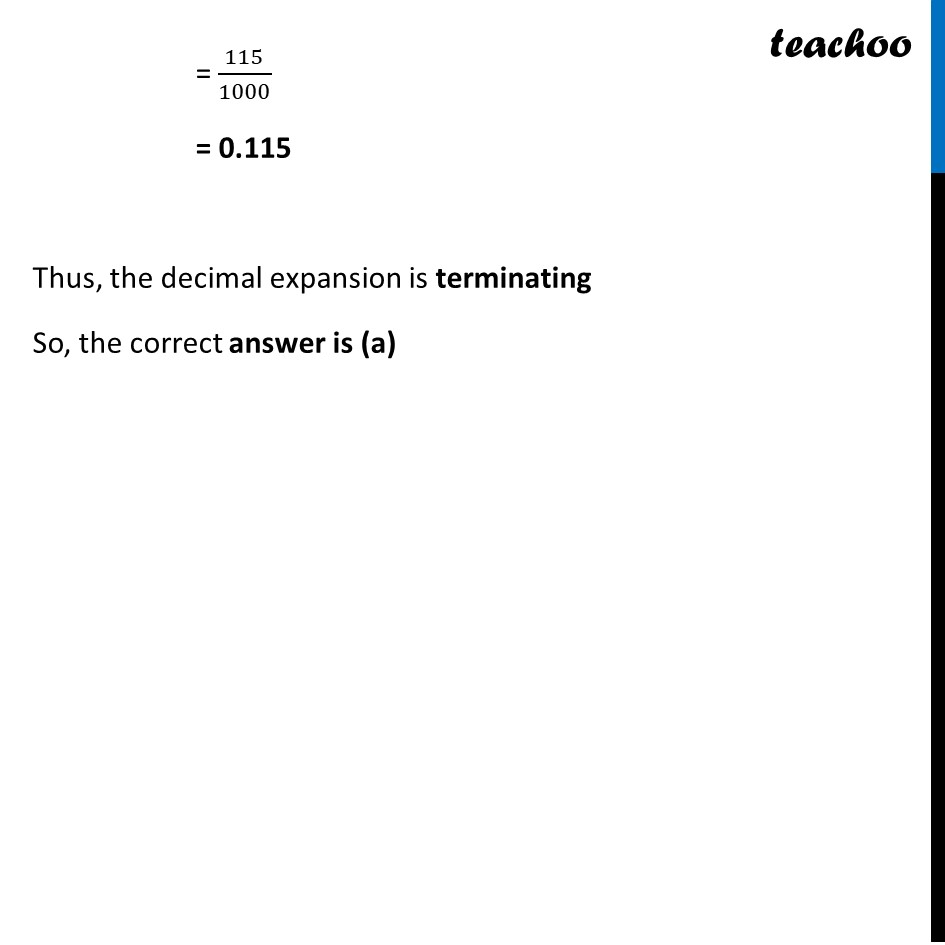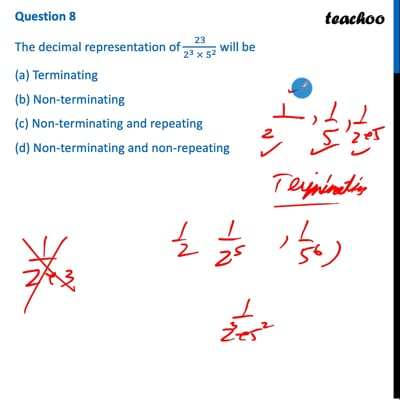CBSE Class 10 Sample Paper for 2022 Boards - Maths Basic [MCQ]

Class 10
Solutions of Sample Papers for Class 10 Boards

## (d) Non-terminating and non-repeatingThis video is only available for Teachoo black users

Introducing your new favourite teacher - Teachoo Black, at only ₹83 per month

### Transcript

Question 8 The decimal representation of 23/(2^3 × 5^2 ) will be (a) Terminating (b) Non-terminating (c) Non-terminating and repeating (d) Non-terminating and non-repeating Now, 𝟐𝟑/(𝟐^𝟑 × 𝟓^𝟐 ) = 23/(2^3 × 5^2 ) × 𝟓/𝟓 = (23 × 5)/(2^3 × 5^3 ) = 115/(2 × 5)^3 = 115/10^3 = 115/1000 = 0.115 Thus, the decimal expansion is terminating So, the correct answer is (a)